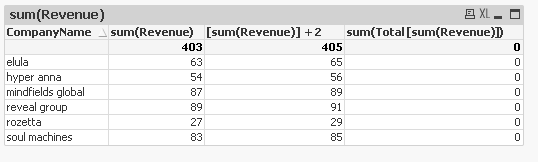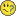New to QlikView

Discussion board where members can get started with QlikView.

New Contributor II

What's wrong with using formula on referenced expressions?

Hi

Can anyone guide me as what's wrong with my expression calculations in following straight table.

Table 1Table 2Here's what's happening,

• I can create some expression based on a metric say Revenue, e.g. sum(Revenue) in Table 1 and max(Revenue) in Table 2.
• Next, I am able to successfully get results with basic operations by referencing this new expression (as [expression] or using labels). For example, [sum(Revenue)] + 2 in Table 1. All good so far• Problems start as soon as I apply any formula to this newly calculated expression and everything goes haywire?As you can see in column 4 of Table 1 and in column 3 of Table 2 above that results are not as expected. The results are wrong no matter what function I apply to the referenced expressions.
• My confusion is that why it's not allowed to apply any formulas to expressions created in charts. Or am i doing something wrong here?Best Regards,

Ehsan

Tags (1)
5 Replies
Honored Contributor II

Re: What's wrong with using formula on referenced expressions?

Sum(TOTAL(Revenue))

Not sure about 2nd example you looking for...

What you like that max function to do?

Revenue should be max of revenue

Valued Contributor

Re: What's wrong with using formula on referenced expressions?

I don't think you are doing anything wrong, just that using the column names like that only works for simple operations (I am happy to be contradicted though). So I would use that to avoid having multiple copies of complex formulae where I then have a difference column (so then you only have to amend the formula in the original column, rather than 2 or more places).

When I look the error message on the formula you have says bad field name - suggests for those more complex calculations it wants to work with actual fields rather than columns you have created.

Regards,

Chris.

New Contributor II

Re: What's wrong with using formula on referenced expressions?

I totally agree and I actually don't refer columns like this.It's just I am using this [ ] referencing here to better explain my queryPartner

Re: What's wrong with using formula on referenced expressions?

-->

=Sum(total(field)) //Sum may not require After total qualifier i guess

-->

There is already an expression as Max(Rev) so =Rev>=(max(rev))  is going to be equal i think.MVP & Luminary

Re: What's wrong with using formula on referenced expressions?

In general you could within a single object refer to another expression by the expression-label or the column(INDEX) - whereby you should rather avoid that the labels are named like existing fields or expressions (it may work in many cases but it could be confusing - for Qlik and yourself - and makes a possible trouble-shooting more difficult). Beside this I'm not sure that you have a label for your column 4 because displaying the expression within the label doesn't mean that a label is existing.

Further you need to add a total-statement within the aggregation if they should ignore all or certain dimension-values like: max(TOTAL Revenue).

For your column 3 you need to add an aggr() if you want that your total is the sum of your rows. This means something like: sum(aggr(sum(Revenue)+2), CompanyName)).

- Marcus